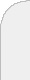inicioir al DIIbuscaron the distance to a geometrical property problems and algorithmic approaches "On the distance to a geometrical property. Problems and algorithmic approaches"We are interested in sets of k points in the n-dimensional space defining a geometrical property, such as -coincidence, collinearity, co-circularity, co-hyperplanarity, linear separability, etc.Given a k-tuple of points in the n-dimensional space, we seek a perturbation vector such that, once perturbed the point satisfies the geometrical property, and the intensity of the perturbation is minimized. The intensity of the perturbation is measured by a nondecreasing function of the vector of norms of the componentwise perturbations.Different geometrical sets as well as different choices of the measure of perturbation yield classical models in different fields such as Operations Research (Facility Location), Machine Learning (Supervised Classification) and Approximation (Nonlinear Regression). Moreover, the optimization problems so obtained have rather different properties, calling for the use of different optimization strategies. We review the state-of-the-art and present new results for some particular instances.